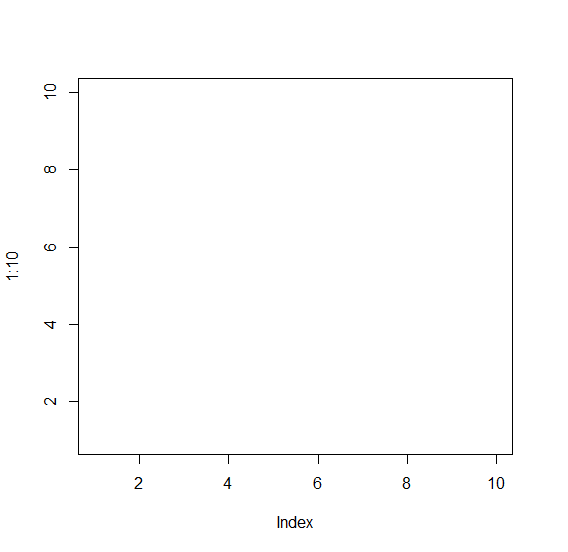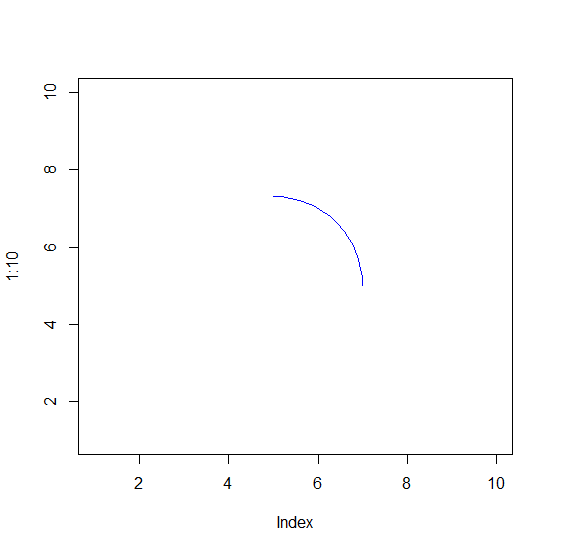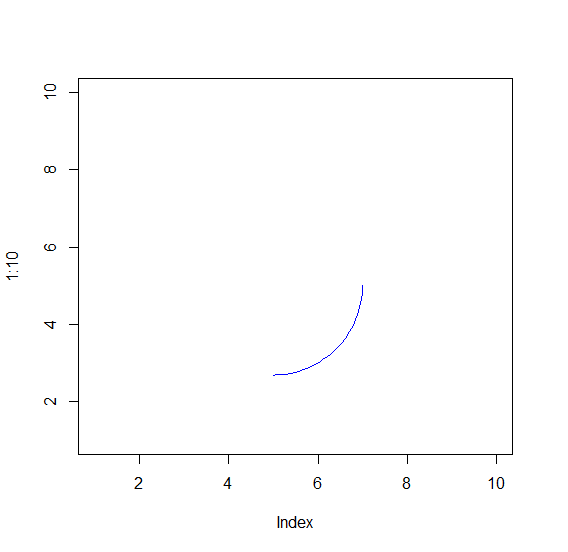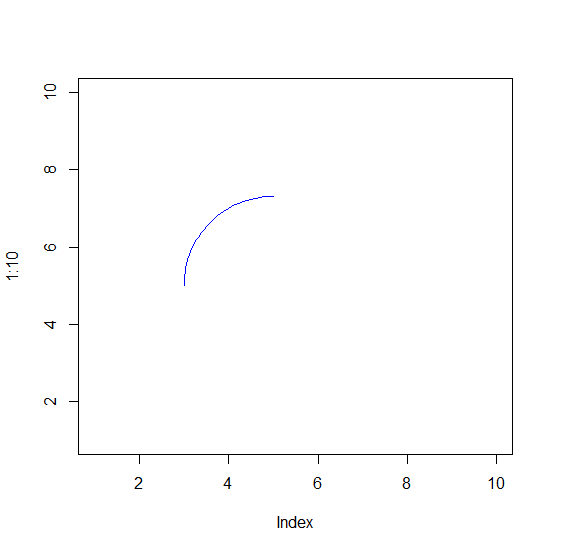# How to create a 90-degree arc in R?

To create a 90-degree arc in R, we can use draw.arc function of plotrix package where we can use deg2 argument. Since, a 90-degree can be drawn in four ways; we need to pass the degree depending on the position of the arc we want to display.

Check out an Example explained below.

## Example

To create a 90-degree arc in R, add the following code −

plot(1:10,type="n")

## Output

If you execute the above given snippet, it generates the following Output −To create a 90-degree arc in R, add the following code to the above snippet −

plot(1:10,type="n")
draw.arc(5,5,2,deg2=90,col="blue")

## Output

If you execute all the above given snippets as a single program, it generates the following Output −To create a 90-degree arc in R, add the following code to the above snippet −

plot(1:10,type="n")
draw.arc(5,5,2,deg2=-90,col="blue")

## Output

If you execute all the above given snippets as a single program, it generates the following Output −To create a 90-degree arc in R, add the following code to the above snippet −

plot(1:10,type="n")
draw.arc(5,5,2,deg1=90,deg2=180,col="blue")

## Output

If you execute all the above given snippets as a single program, it generates the following Output −